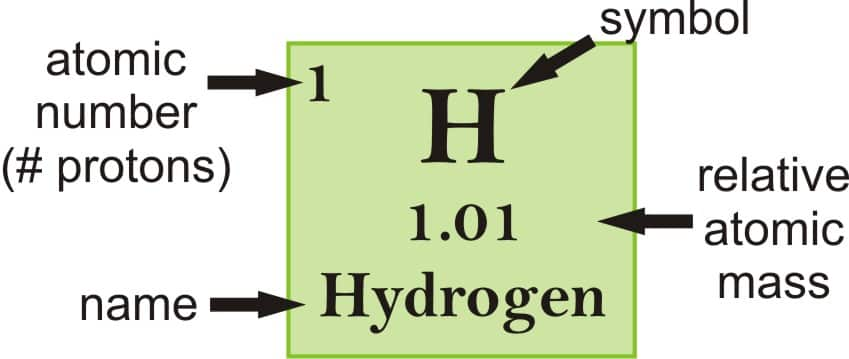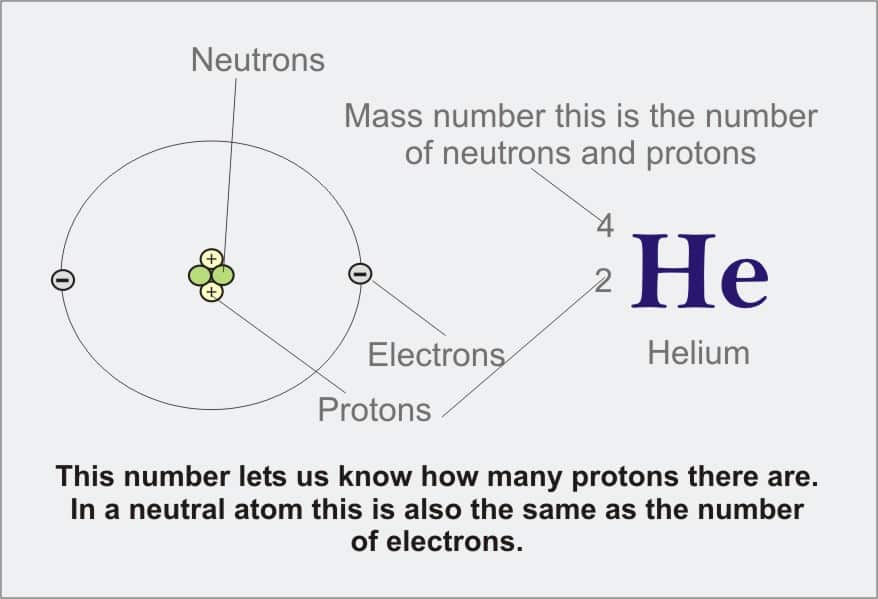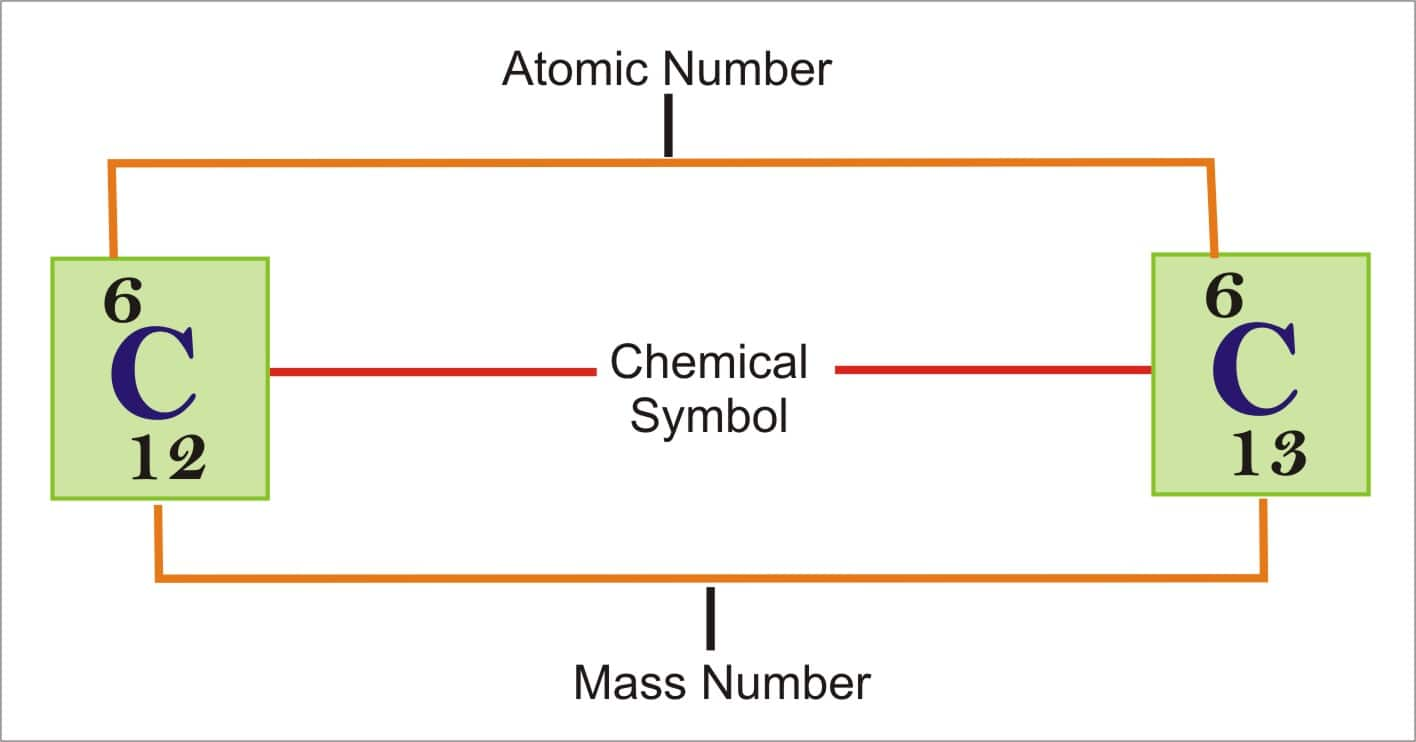# Atomic Number and Mass Number

## Difference Between Atomic Number and Mass Number

The current periodic table is set in such a way that all the elements have an increasing atomic number, and consequently, increasing mass number. But do we know what mass number, or even what atomic number is? Well, as we know, an atom comprises of electrons, protons, and neutrons. The number of electrons in the outer most shells gives us the valency of the atom. In the same way, the number of protons and neutrons are related to the atomic number and mass number of the atom.

Atomic number, atomic mass, and relative atomic mass

Atoms of each element consist of a particular number of protons. In fact, the number of protons dictates what atom we are looking at (e.g., all atoms with 6 protons are carbon atoms). Atomic number is the number of protons there in an atom. On the other hand, the number of neutrons for a given element can differ. Together, the number of protons and the number of neutrons dictates an element’s mass number.

Mass number = Number of protons + Number of neutrons

If you want to calculate the number of neutrons in an atom, you can simply deduct the number of protons, or atomic number, from the mass number.

A property strongly related to an atom’s mass number is its atomic mass. The atomic mass of a single atom is basically its total mass and is usually expressed in atomic mass units or AMU. By definition, an atom of carbon with 6 neutrons, carbon-12, has an atomic mass of 12 amu. In general, an atom's atomic mass will be very close to its mass number but will have some difference in the decimal places.

An isotope of an element is a variant of that particular element which consists of a different in the number of neutron and further in the nucleon number. All isotopes of a particular element have exactly the same number of protons but again only differ in the number of neutrons in each atom. Since an element’s isotope differs in their atomic masses, scientists may also establish the relative atomic mass at times called the atomic weight for an element. The relative atomic mass is an average of all the atomic masses of different isotopes in a given sample, with each isotope's contribution to the average.

The relative atomic masses specified in periodic table entries—for example, hydrogen—are calculated for all the naturally occurring isotopes of every element, weighted by the quantity of those isotopes on earth. Objects, like asteroids or meteors, might have very different isotope quantity.Atomic Number

The atomic number (expressed by the letter Z) of an element is the number of protons in the nucleus of each atom of that element. An atom can be classified as an element based exclusively on its atomic number. For instance, an atom with an atomic number of eight (its nucleus contains 8 protons) is an oxygen atom, and an atom with a different number of protons would be a different element. The periodic table is set in an order of increasing atomic number.

Since atoms are neutral, the number of protons in an atom is equal to the number of electrons. Hydrogen atoms have 1 electron taking up space outside of the nucleus. Helium, which consists of 2 protons, will have 2 electrons due to the neutral state. In the chemical classification, the proton count will always be equal to an atom's atomic number and this value will not be subjected to any changes unless the nucleus undergoes decay causing the atom to lose its energy; also called radioactive decay.

Importance of Atomic Numbers

• • Helps in the identification of a selected element of an atom.

• • The arrangement of the elements is done on the basis of the atomic number. The elements are set in increasing order of the atomic numbers of the elements.

• • Helps in the discovery of the properties of any element. However, the valence electron decides the chemical bonding behavior of an element.

•  Examples of Atomic Numbers

It doesn’t matter how many protons and electrons are present in an atom. Atomic number is always calculated by the number of protons. For instance,

• • If an atom has 1 proton then the atomic number is 1 and the element is hydrogen.

• • Every carbon atom will have six protons and the atomic number of carbon is 6.

• • Every cesium atom has 55 protons and therefore, the atomic number is 55.

• Atomic numbers of few elements:

• • Hydrogen= 1

• • Helium=2

• • Lithium=3

• • Beryllium=4

• • Boron=5

• • Carbon=6

• • Nitogen=7

• • Oxygen=8

• Finding the Atomic Number

An atomic number calculated as follows:

• • Firstly, an atomic number can be determined by the number of protons in an atom of an element.

• • Secondly, if an element name or symbol is given then it can be recognized by using a periodic table chart. It is a simple positive and whole number which is denoted by the letter Z. For instance, if the symbol Al (aluminium) is given. If you explore the periodic table you can notice the atomic number of Al is 13. It is easy to find out in a periodic table since the elements are present in increasing order.

•• •
• Thirdly, it can be recognized by the isotope symbol. Isotope symbol can be written in a lot of ways but the symbol is always given while writing an isotope. Such as, if an isotope of carbon is given as 14C or 146C. 14C- If the isotope is written in such a manner, we can identify that the symbol represents carbon atom and the atomic number of carbon atom is 6. 146C- If the isotope is written in such a manner, the atomic number will be the whole number and smaller of the two numbers which is 6. It is generally the subscript of the element symbol.

• Mass Number

An element’s mass number (A) is the total of the number of protons and the number of neutrons. The small role of mass from electrons is overlooked in calculating the mass number. This approximation of mass can be used to easily determine how many neutrons an element has by simply deducting the number of protons from the mass number. Isotopes of the same element will have a similar atomic number but different mass numbers.

Carbon has an atomic number of 6, and 2 stable isotopes with mass numbers of 12 and 13, respectively. Its average atomic mass is 12.11.

Scientists calculate the atomic mass by averaging the mass numbers for its naturally-occurring isotopes. Frequently, the resulting number contains a decimal. For instance, the atomic mass of chlorine (Cl) is 35.45 amu because chlorine is made of several isotopes, some having an atomic mass of 35 amu and some with an atomic mass of 37 amu.

With the help of an atomic number (Z) and mass number (A), you can find the number of protons, neutrons, and electrons in a neutral atom. For instance, a lithium atom (Z=3, A=7 amu) has 3 protons (found from Z), 3 electrons (as the number of protons is equivalent to the number of electrons in an atom), and 4 neutrons (7 – 3 = 4).

Use of Mass Number

The mass number of an element helps to determine the isotopic mass which is measured in the atomic mass units or “U”.  An isotope of an element will consist of a similar atomic number but a different mass number. Isotope mostly differs in the number of neutrons. Different isotopes of the same element will not have the same mass number. Though, the isotopes of different elements can have the same mass number such as carbon-14 (6 protons + 8 neutrons) and nitrogen-14 (7 protons + 7 neutrons).

Properties of Mass Number

The diverse properties of mass number are listed below. Let us have a look,

• • Total of protons and neutrons provide this number of a certain element.

• • It is represented by the letter A.

• • Neutrons and Protons are together are termed as nucleons.

• • Example: Atoms of carbon comprises of 6 protons and 6 neutrons. Hence, the mass number of Carbon is 12.

• • The number of neutrons may differ in an element. Although, the total number of protons is the same in all atoms of an element. Hence, the atoms of the same element with the same atomic number but a different mass number are termed as isotopes.

• • Normally, atomic mass and mass numbers are two different terms and may differ slightly. In most cases, they are not the same. However, the weight of an electron is very less so we can believe the atomic mass of an atom to be almost equal to its mass number.

•There are some substances called Isobars. These are atoms of different elements that have the same mass number, but they have different atomic numbers. For instance, Chlorine-37 and Argon-37 have the same mass number. Hence, they are isobars.

The atoms often experience alpha decay that destroys 2 protons and 2 neutrons from a radioactive nucleus, the mass number of elements can be altered accordingly. Finally, the mass number is changed by 4 units.

Difference between Atomic number and Mass Number

Atomic Number: The number of protons present in an atom is known as Atomic number.

Mass Number:  Mass number is the sum of the number of neutrons and protons of an atom.

Number of Neutrons

Atomic Number:  The atomic number of an atom is not affected by the number of neutrons.

Mass Number: The mass number of an atom is not affected by the number of neutrons.

Isotopes

Atomic Number: Isotopes have a similar atomic number.

Mass Number
: Isotopes have different mass numbers.

Isobars

Atomic Number: The atomic number in isobars cannot be the same.

Mass Number: The mass number in isobars can be the same.

Value

Atomic Number: The atomic number is always a lesser value than the mass number.

Mass Number: The mass number is always a greater value than the atomic number.

Atoms are made of electrons, protons, and neutrons. Protons and neutrons together form the nucleus of an atom. While measuring the mass of an atom, we actually measure the mass of the nucleus. That is because the mass of an electron is very less when compared to a proton or a neutron. The atomic number and the mass number of an atom are two main terms because these terms provide us with facts about the number of protons and the number of neutrons present in an atom. The major difference between atomic number and mass number is that the atomic number states the number of protons present in an atom whereas, the mass number indicates the total of the number of protons and the number neutrons present in an atom.